﻿ 基于车内外视觉信息的行人碰撞预警方法
«上一篇文章快速检索 高级检索

 智能系统学报2019, Vol. 14Issue (4): 752-760  DOI: 10.11992/tis.2018010160

### 引用本文YANG Huicheng, ZHU Wenbo, TONG Ying. Pedestrian collision warning system based on looking-in and looking-out visual information analysis[J]. CAAI Transactions on Intelligent Systems, 2019, 14(4): 752-760. DOI: 10.11992/tis.201801016.### 文章历史

Pedestrian collision warning system based on looking-in and looking-out visual information analysis
YANG Huicheng , ZHU Wenbo, TONG Ying
College of Electrical Engineering, Anhui Polytechnic University, Wuhu 241000, China
Abstract: Pedestrian collision warning systems usually provide early warning for drivers based on the technologies of pedestrian detection and collision time measurement. To provide a more reliable basis for risk assessment, a pedestrian collision warning method that involves analyzing the road condition and driver’s head pose simultaneously is proposed in this paper. Two monocular cameras are used to capture vehicle exterior and interior images, and a channel features detector is applied to locate pedestrians. The vertical and horizontal distances between pedestrians and ego-vehicle are estimated based on monocular vision distance measurement. The multi-task cascaded convolutional network is utilized for facial landmark detection. By solving a perspective-n-point (PnP) problem, the estimated head angles can reflect driver’s attention states. By combining both pedestrian location information and driver’s attention information, we implemented a fuzzy inference system to assess collision risk level. An experiment in real-world driving conditions demonstrated that the risk levels obtained from the fuzzy system are reliable and can provide guidance for collision avoidance.
Key words: collision warning    internal and external information    pedestrian positioning    driver states    monocular vision    channel features    multi-task cascaded convolutional network    fuzzy inference system

1 行人位置估计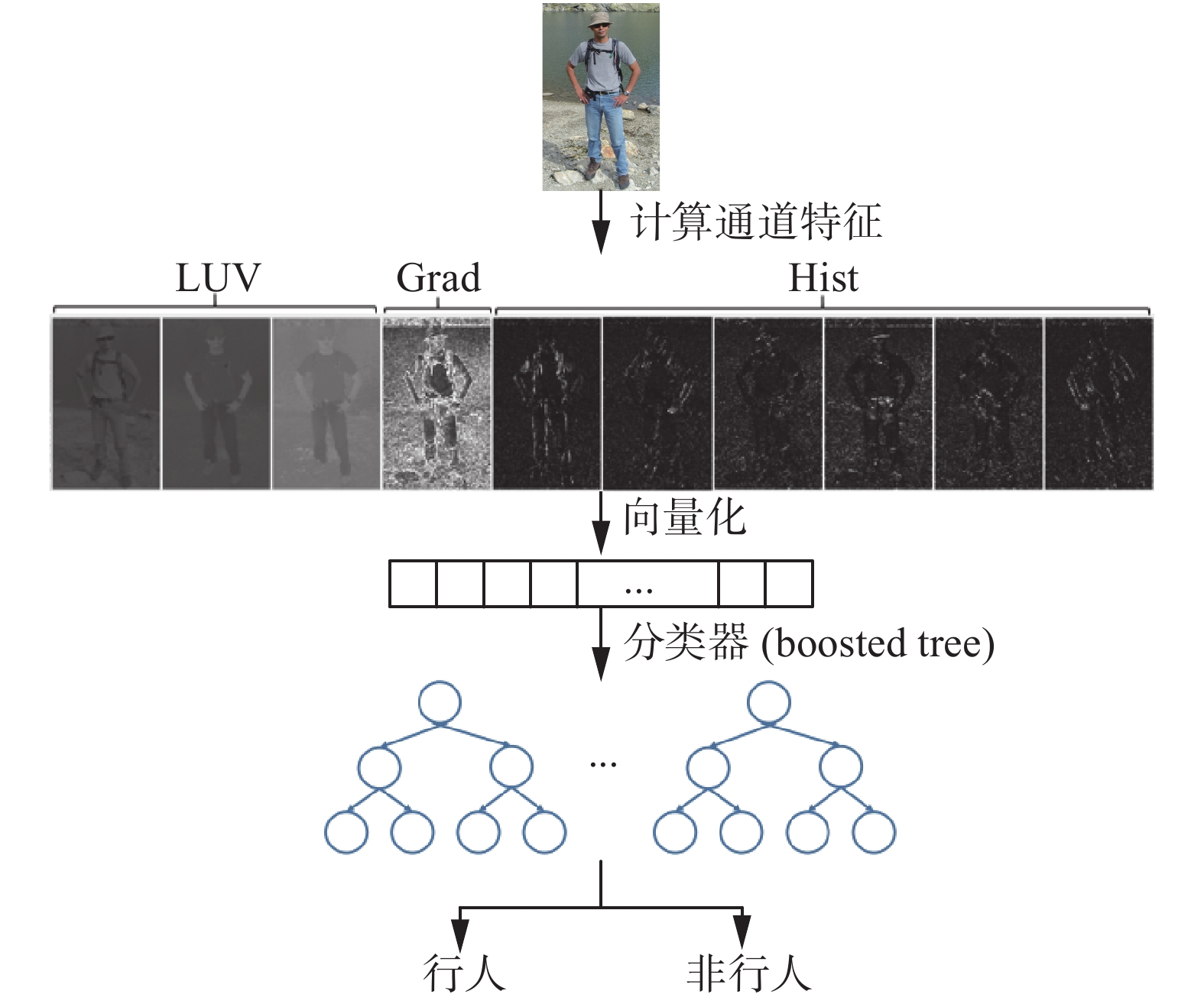Download: 图 1 通道特征检测器 Fig. 1 Channel features detector

 ${C_s} \approx R(C,s) \cdot {s^{ - {\lambda _\varOmega }}}$ (1)

 ${C_{s'}} = R({C_s},{s/{s'}}){({s/{s'}})^{ - {\lambda _\varOmega }}},\;\;s' \in \{ 1,\frac{1}{2},\frac{1}{4}, \cdots \}$ (2)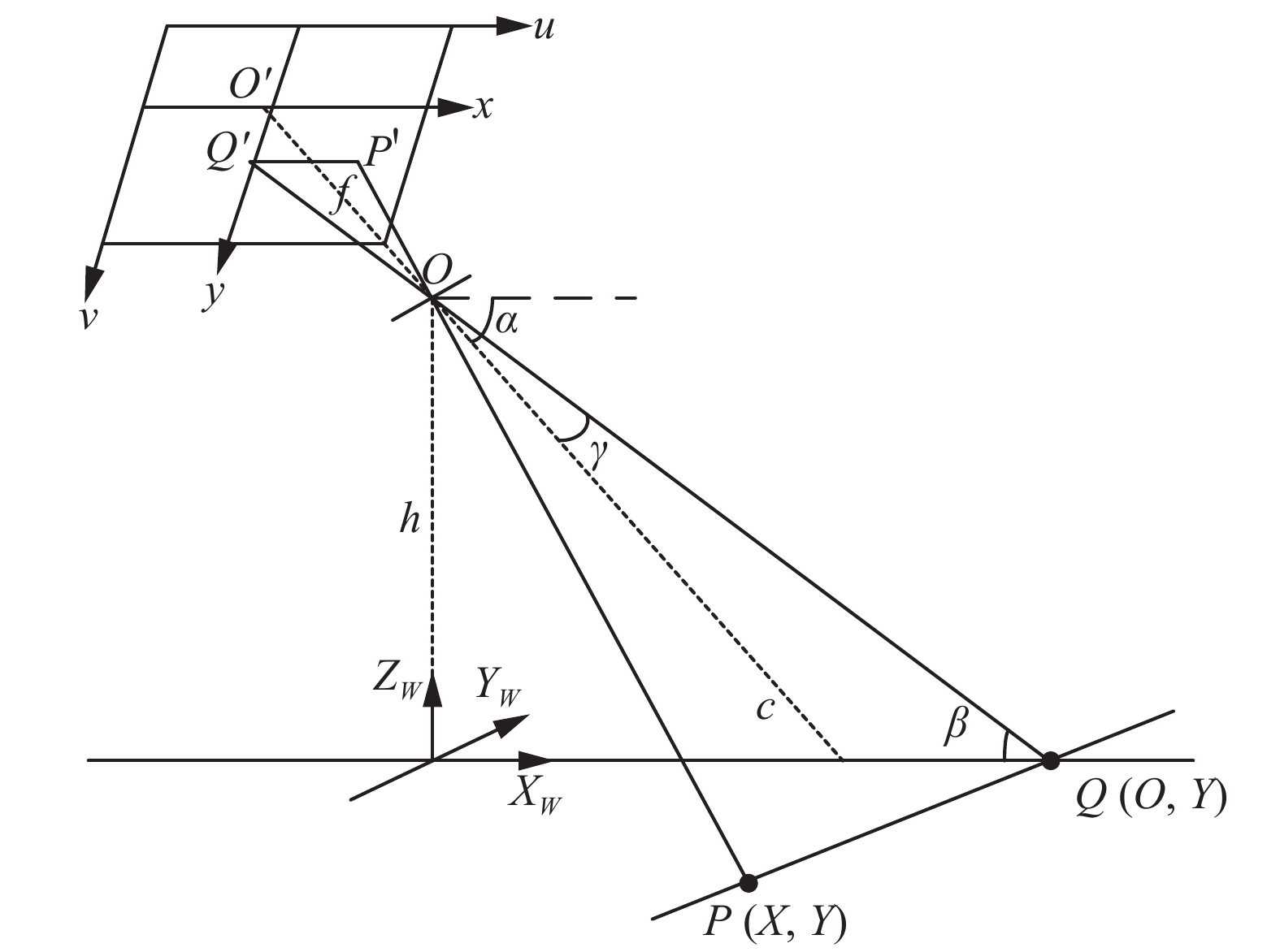Download: 图 2 单目视觉距离估计模型 Fig. 2 Monocular vision distance estimation model

 $Y = \frac{h}{{\tan \beta }} = \frac{h}{{\tan (\alpha - {\rm arctan} \displaystyle\frac{y}{f})}}$ (3)

 $\begin{gathered} X = \frac{{P'Q'}}{{OQ'}}Y = \frac{x}{{\sqrt {{f^2} + {y^2}} }}\frac{h}{{\sin (\alpha - {\rm arctan} \displaystyle\frac{y}{f})}}{\rm{ = }} \\ \;\;\;\frac{{(u - {u_0})h}}{{\sqrt {{f^2} + {{(v - {v_0})}^2}} \sin (\alpha - {\rm arctan} \displaystyle\frac{y}{f})}} \end{gathered}$ (4)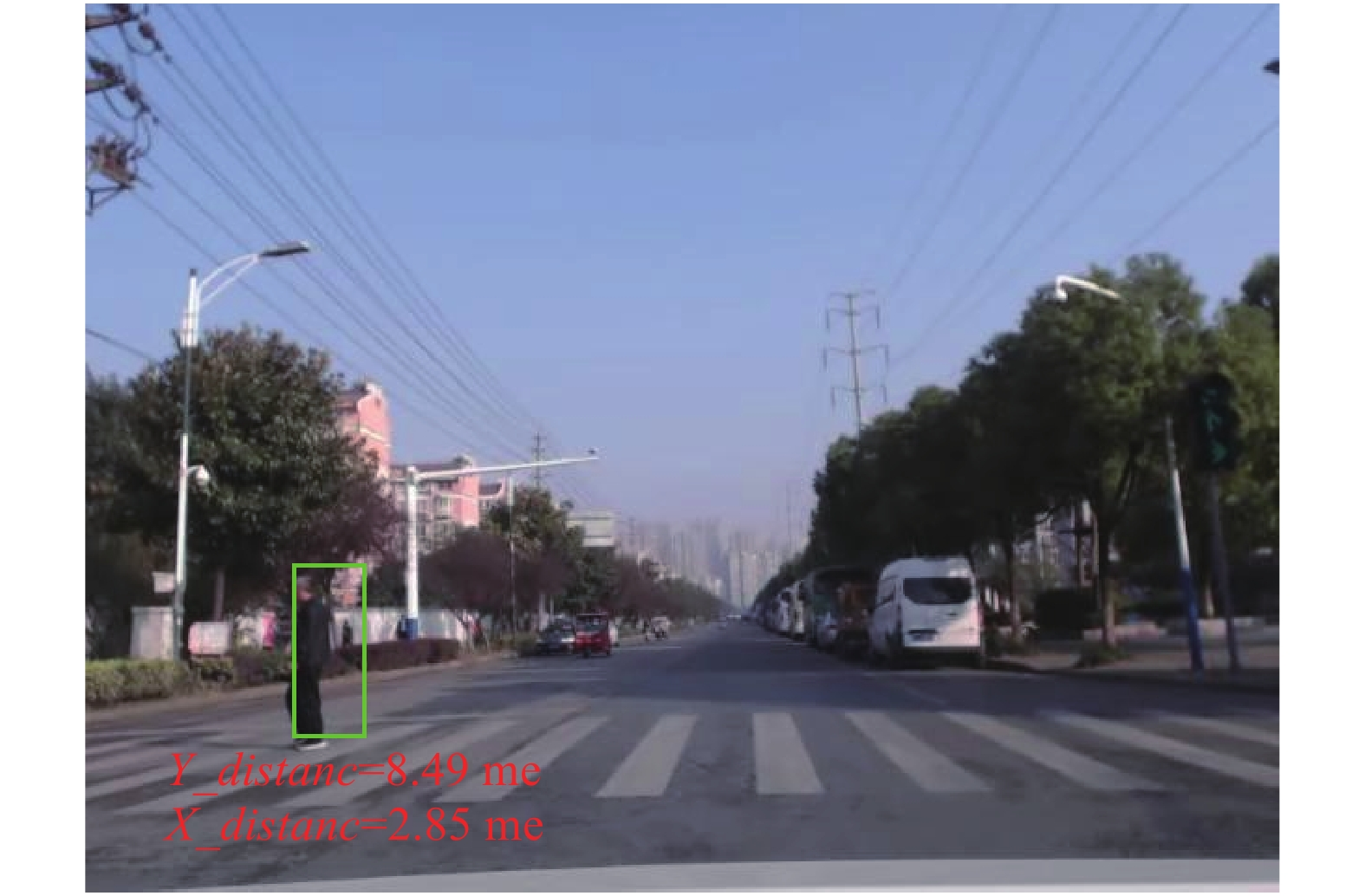Download: 图 3 行人检测与距离估计结果 Fig. 3 Pedestrian detection and distance estimation
2 驾驶员头部姿态估计

 $\left[ {\begin{array}{*{20}{c}} \!\!\! X \!\!\! \\ \!\!\! Y \!\!\! \\ \!\!\! Z \!\!\! \end{array}} \right] = \left[ {{ R}\left| {\;{ T}} \right.} \right]\left[ {\begin{array}{*{20}{c}} \!\!\! U \!\!\! \\ \!\!\! V \!\!\! \\ \!\!\! W \!\!\! \\ 1 \end{array}} \right],\;\;{ R} = \left[ {\begin{array}{*{20}{c}} \!\!\! {{r_{11}}}&{{r_{12}}}&{{r_{13}}} \!\!\! \\ \!\!\! {{r_{21}}}&{{r_{22}}}&{{r_{23}}} \!\!\! \\ \!\!\! {{r_{31}}}&{{r_{32}}}&{{r_{33}}} \!\!\! \end{array}} \right],\;\;{ T} = \left[ {\begin{array}{*{20}{c}} \!\!\! {{t_x}} \!\!\! \\ \!\!\! {{t_y}} \!\!\! \\ \!\!\! {{t_z}} \!\!\! \end{array}} \right]$ (5)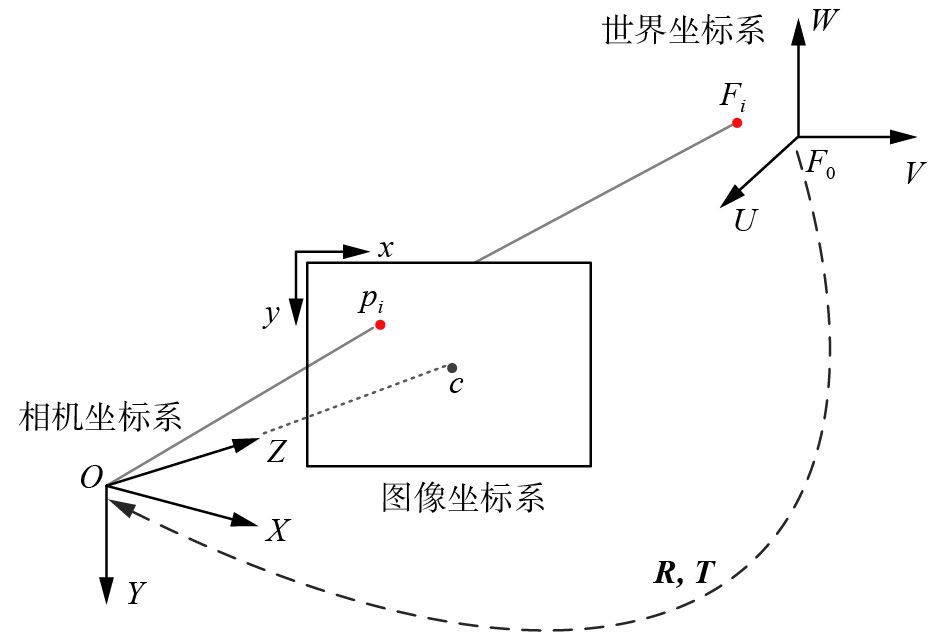Download: 图 4 头部三维模型到图像平面特征点的投影 Fig. 4 Projection of 3D model of the head to the feature points of image plane

 ${F_i} = \sum\limits_{j = 1}^4 {{\alpha _{ij}}c_j^F} ,\;\;\sum\limits_{j = 1}^4 {{\alpha _{ij}} = 1}$ (6)

 ${p_i} = \sum\limits_{j = 1}^4 {{\alpha _{ij}}c_j^p}$ (7)

 ${\omega _n}\left[ {\begin{array}{*{20}{c}} {{u_i}} \\ {{v_i}} \\ 1 \end{array}} \right] = \left[ {\begin{array}{*{20}{c}} {{f_x}}&0&{{c_x}} \\ 0&{{f_y}}&{{c_y}} \\ 0&0&1 \end{array}} \right]\sum\limits_{j = 1}^4 {{\alpha _{ij}}} \left[ {\begin{array}{*{20}{c}} {x_j^p} \\ {y_j^p} \\ {z_j^p} \end{array}} \right]$ (8)

 $\left\{ {\begin{array}{*{20}{c}} {\displaystyle\sum\limits_{j = 1}^4 {{\alpha _{ij}}{f_x}x_j^p + {\alpha _{ij}}({c_x} - {u_i})z_j^p} = 0} \\ {\displaystyle\sum\limits_{j = 1}^4 {{\alpha _{ij}}{f_y}x_j^p + {\alpha _{ij}}({c_y} - {v_i})z_j^p} = 0} \end{array}} \right.$ (9)

 $\left\{ {\begin{array}{l} {{\rm pitch} = {\rm{arctan}} 2({r_{32}},\;{r_{33}})} \\ {{\rm yaw} = {\rm{arctan}} 2( - {r_{31}},\;\sqrt {r_{32}^2 + r_{33}^2} )} \\ {{\rm roll} = {\rm{arctan}} 2({r_{21}},\;{r_{11}})} \end{array}} \right.$ (10)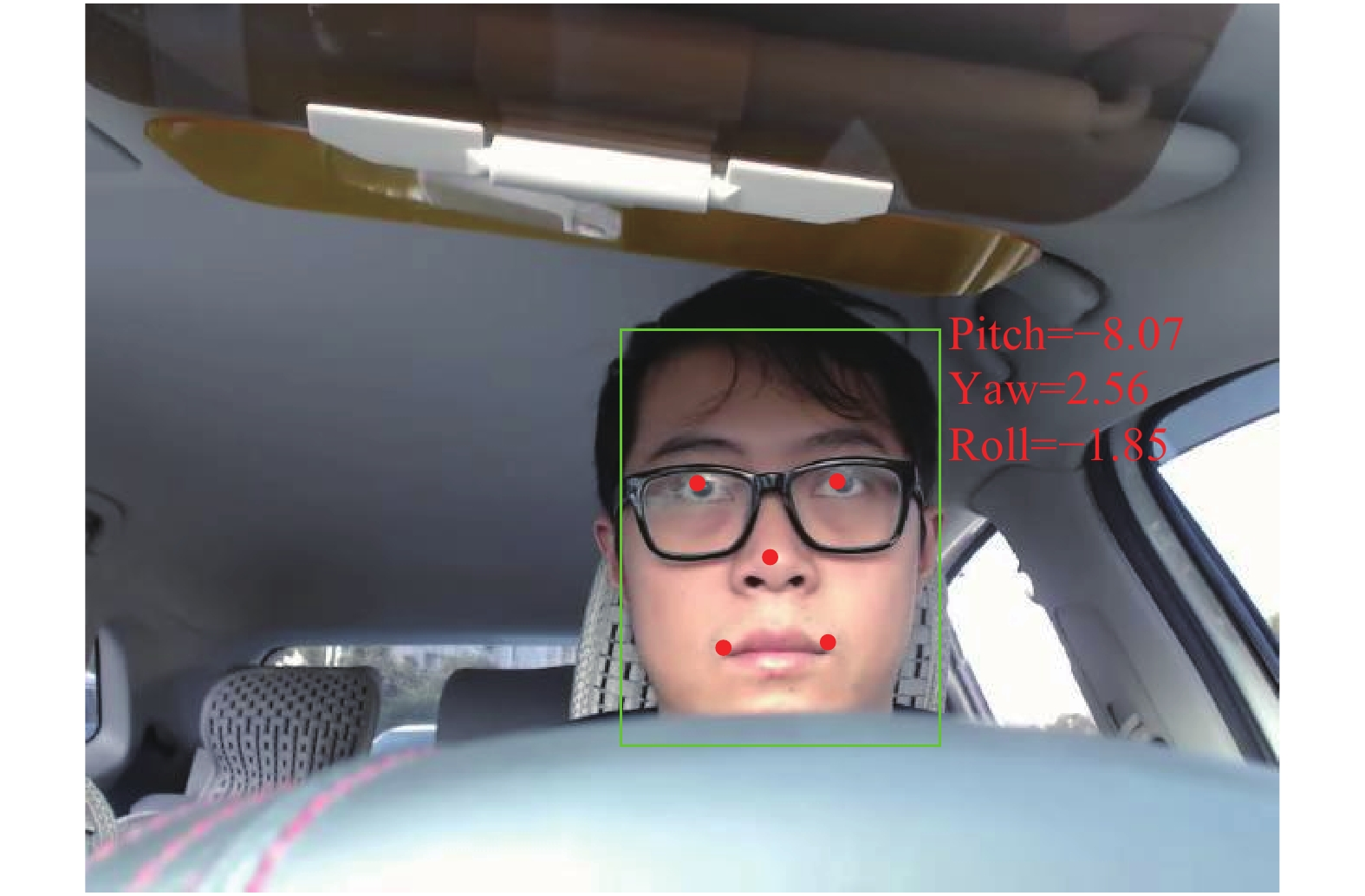Download: 图 5 驾驶员头部姿态估计 Fig. 5 Head pose estimation of the driver
3 碰撞风险评估

 $\begin{gathered} {R^i}:\;{\rm if}\;{x_1}\;{\rm is}\;A_1^i,\;{x_2}\;{\rm is}\;A_2^i,\; \cdots ,\;{x_k}\;{\rm is}\;A_k^i \\ \;\;\;\;\;\;\;\;\;\;\;\;\;\;\;{\rm then}\;{y^i} = {B^i} \\ \end{gathered}$ (11)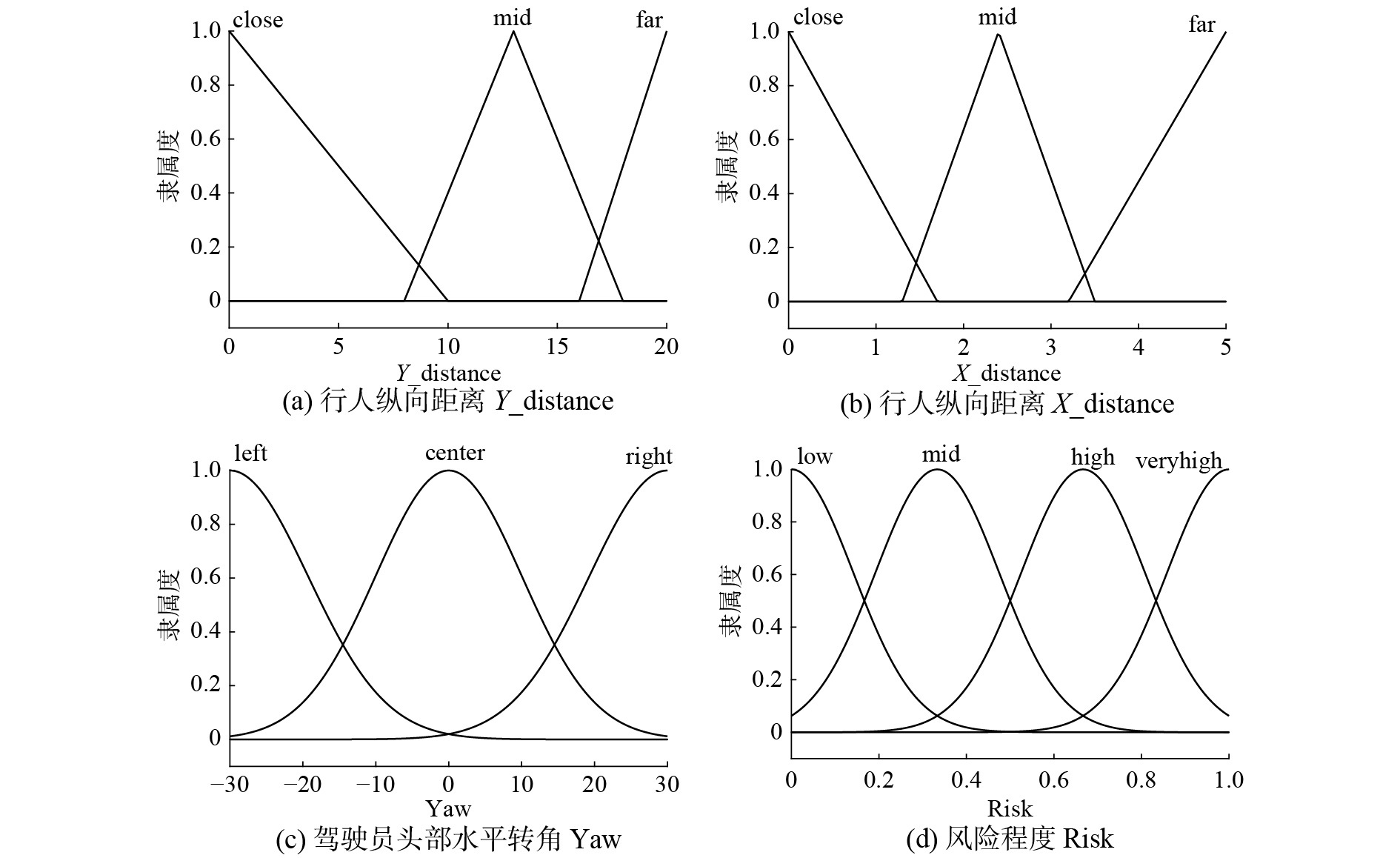Download: 图 6 模糊系统输入输出隶属度函数 Fig. 6 Input/output membership functions of fuzzy system表 1 模糊推理规则 Tab.1 Fuzzy inference rules
4 实验分析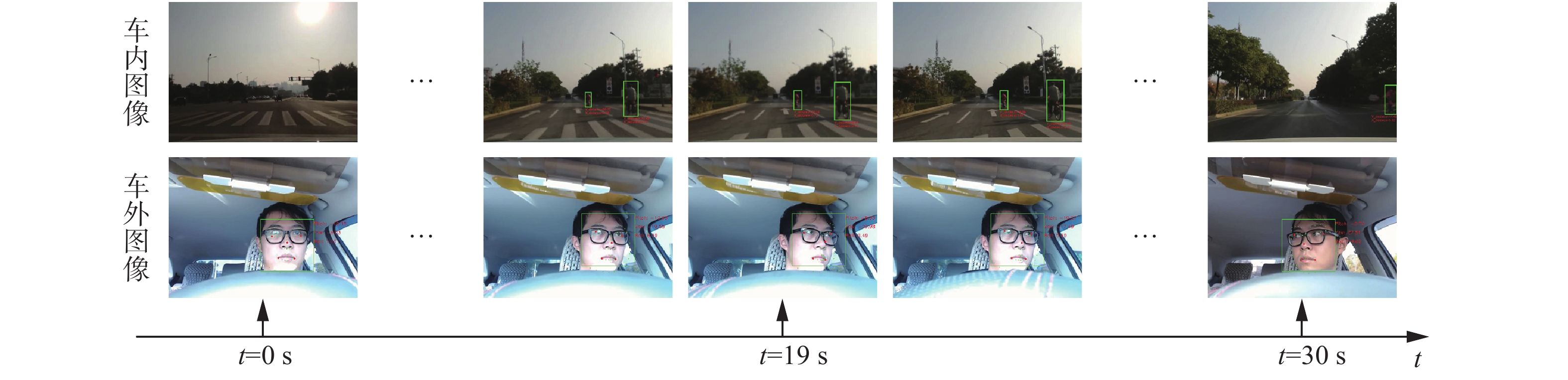Download: 图 7 视频不同时间点采集的车内外图像 Fig. 7 Interior and exterior images collected at different time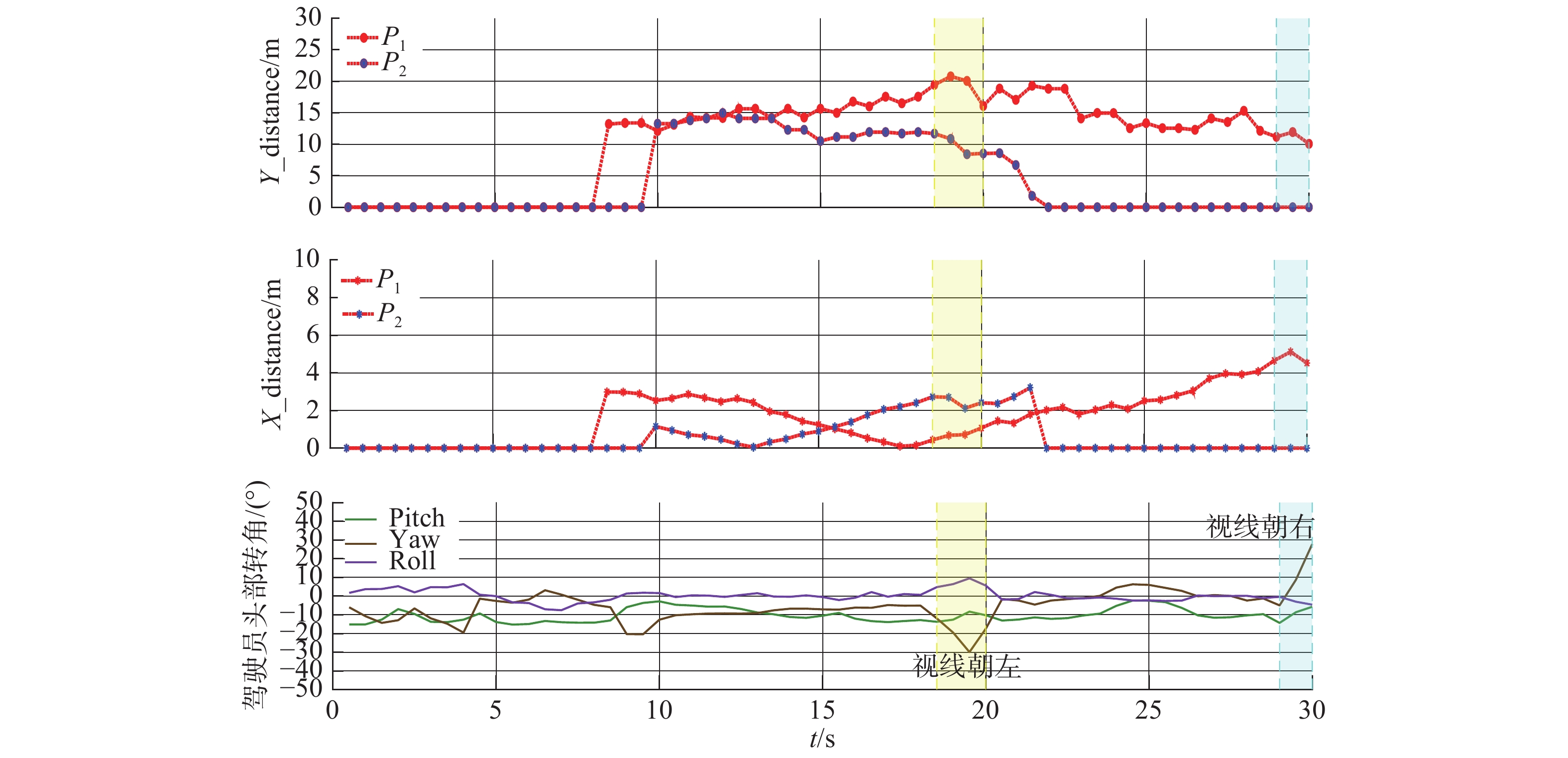Download: 图 8 行人与驾驶员状态数据 Fig. 8 Data of pedestrian and driver status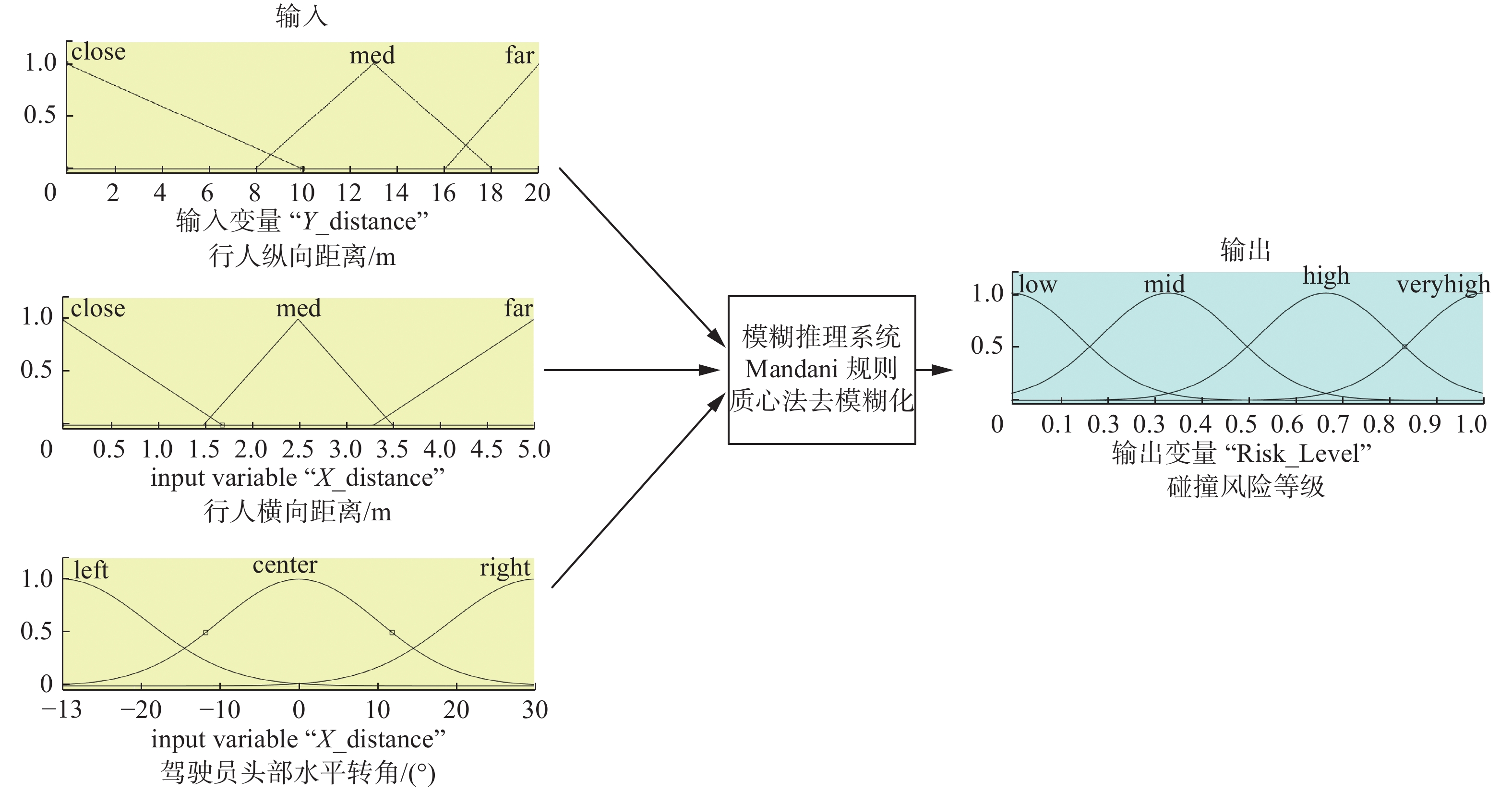Download: 图 9 3个输入(Y_distance, X_distance, Yaw)的模糊推理系统 Fig. 9 Fuzzy inference system of three inputs (Y_distance, X_distance, Yaw)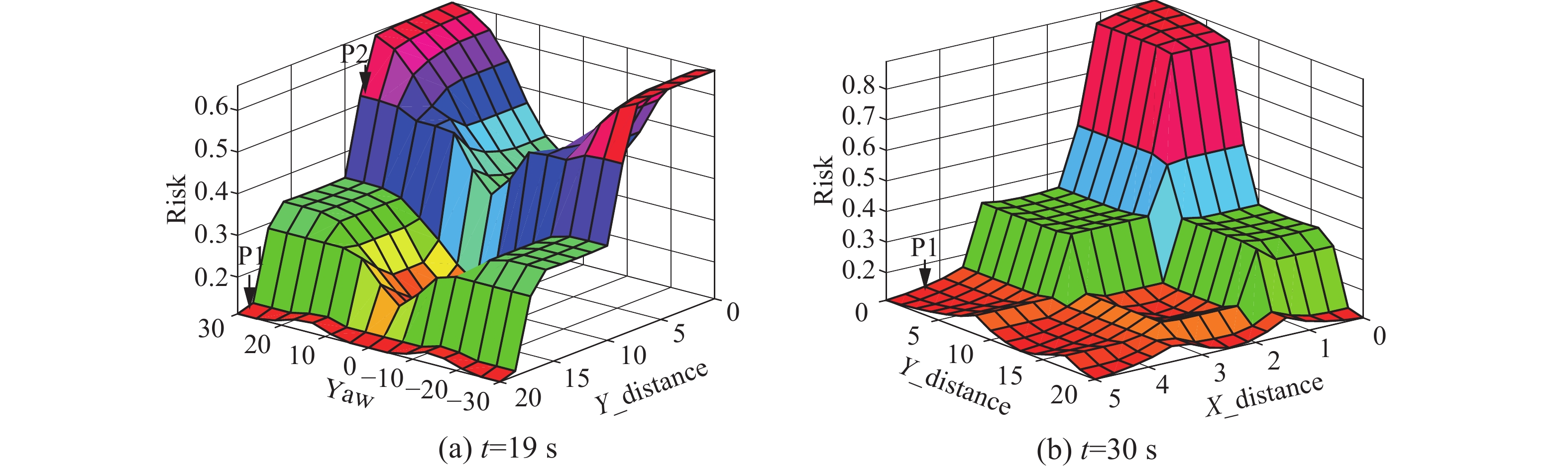Download: 图 10 碰撞风险等级的解模糊平面 Fig. 10 Defuzzified surface of the collision risk level

5 结束语

  VIOLA P, JONES M. Rapid object detection using a boosted cascade of simple features[C]//Proceedings of 2001 IEEE Computer Society Conference on Computer Vision and Pattern Recognition. Kauai, USA, 2001: I-511-I-518. (0)  DALAL N, TRIGGS B. Histograms of oriented gradients for human detection[C]//Proceedings of 2005 IEEE Computer Society Conference on Computer Vision and Pattern Recognition. San Diego, USA, 2005: 886–893. (0)  FELZENSZWALB P F, GIRSHICK R B, MCALLESTER D, et al. Object detection with discriminatively trained part-based models[J]. IEEE Transactions on pattern analysis and machine intelligence, 2010, 32(9): 1627-1645. DOI:10.1109/TPAMI.2009.167 (0)  GIRSHICK R, DONAHUE J, DARRELL T, et al. Rich feature hierarchies for accurate object detection and semantic segmentation[C]//Proceedings of 2014 IEEE Conference on Computer Vision and Pattern Recognition. Columbus, USA, 2014: 580–587. (0)  GIRSHICK R. Fast R-CNN[C]//Proceedings of 2015 IEEE International Conference on Computer Vision. Santiago, USA, 2015: 1440–1448. (0)  STANTON N A, SALMON P M. Human error taxonomies applied to driving: a generic driver error taxonomy and its implications for intelligent transport systems[J]. Safety science, 2009, 47(2): 227-237. DOI:10.1016/j.ssci.2008.03.006 (0)  TAWARI A, MARTIN S, TRIVEDI M M. Continuous head movement estimator for driver assistance: issues, algorithms, and on-road evaluations[J]. IEEE transactions on intelligent transportation systems, 2014, 15(2): 818-830. DOI:10.1109/TITS.2014.2300870 (0)  COOTES T F, EDWARDS G J, TAYLOR C J. Active appearance models[J]. IEEE transactions on pattern analysis and machine intelligence, 2001, 23(6): 681-685. DOI:10.1109/34.927467 (0)  REN Shaoqing, CAO Xudong, WEI Yichen, et al. Face alignment at 3000 fps via regressing local binary features[C]//Proceedings of 2014 IEEE Conference on Computer Vision and Pattern Recognition. Columbus, USA, 2014: 1685–1692. (0)  SUN Yi, WANG Xiaogang, TANG Xiaoou. Deep convolutional network cascade for facial point detection[C]//Proceedings of 2013 IEEE Conference on Computer Vision and Pattern Recognition. Portland, USA, 2013: 3476–3483. (0)  ZHANG Kaipeng, ZHANG Zhanpeng, LI Zhifeng, et al. Joint face detection and alignment using multitask cascaded convolutional networks[J]. IEEE Signal processing letters, 2016, 23(10): 1499-1503. DOI:10.1109/LSP.2016.2603342 (0)  DOLLÁR P, APPEL R, BELONGIE S, et al. Fast feature pyramids for object detection[J]. IEEE transactions on pattern analysis and machine intelligence, 2014, 36(8): 1532-1545. DOI:10.1109/TPAMI.2014.2300479 (0)  BOURDEV L, BRANDT J. Robust object detection via soft cascade[C]//Proceedings of 2005 IEEE Computer Society Conference on Computer Vision and Pattern Recognition. San Diego, USA, 2005: 236–243. (0)  王牛, 李祖枢, 武德臣, 等. 机器人单目视觉定位模型及其参数辨识[J]. 华中科技大学学报(自然科学版), 2008, 36(S1): 57-60. WANG Niu, LI Zushu, WU Dechen, et al. Robot monocular vision position determination model and its parametric identification[J]. Journal of Huazhong University of Science and Technology (Nature Science Edition), 2008, 36(S1): 57-60. (0)  LEPETIT V, MORENO-NOGUER F, FUA P. EPnP: an accurate O(n) solution to the PnP problem [J]. International journal of computer vision, 2009, 81(2): 155-166. DOI:10.1007/s11263-008-0152-6 (0)  TAWARI A, SIVARAMAN S, TRIVEDI M M, et al. Looking-in and looking-out vision for Urban Intelligent Assistance: estimation of driver attentive state and dynamic surround for safe merging and braking[C]//Proceedings of 2014 IEEE Intelligent Vehicles Symposium. Dearborn, USA, 2014: 115–120. (0)  TATEIWA K, YAMADA K. Estimating driver awareness of pedestrians in crosswalk in the path of right or left turns at an intersection from vehicle behavior[C]//Proceedings of 2015 IEEE Intelligent Vehicles Symposium. Seoul, South Korea, 2015: 952–957. (0)  ROTH M, FLOHR F, GAVRILA D M. Driver and pedestrian awareness-based collision risk analysis[C]//Proceedings of 2016 IEEE Intelligent Vehicles Symposium. Gothenburg, Sweden, 2016: 454–459. (0)